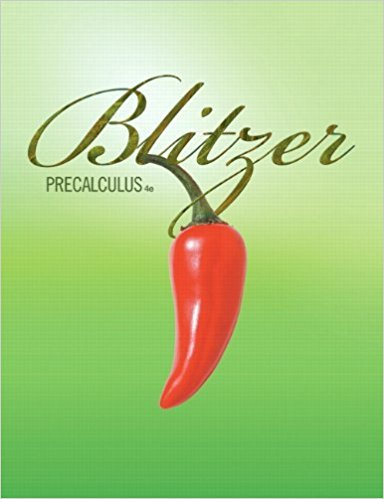×
Log in to StudySoup
Get Full Access to Calculus - Textbook Survival Guide
Join StudySoup for FREE
Get Full Access to Calculus - Textbook Survival Guide

Already have an account? Login here
×
Reset your password

# Solutions for Chapter 6.7: The Dot Product## Full solutions for Precalculus | 4th Edition

ISBN: 9780321559845Solutions for Chapter 6.7: The Dot Product

Solutions for Chapter 6.7
4 5 0 380 Reviews
19
1
##### ISBN: 9780321559845

Chapter 6.7: The Dot Product includes 89 full step-by-step solutions. Since 89 problems in chapter 6.7: The Dot Product have been answered, more than 107663 students have viewed full step-by-step solutions from this chapter. This textbook survival guide was created for the textbook: Precalculus, edition: 4. This expansive textbook survival guide covers the following chapters and their solutions. Precalculus was written by and is associated to the ISBN: 9780321559845.

Key Calculus Terms and definitions covered in this textbook
• Angle

Union of two rays with a common endpoint (the vertex). The beginning ray (the initial side) can be rotated about its endpoint to obtain the final position (the terminal side)

• Components of a vector

See Component form of a vector.

• Coordinate plane

See Cartesian coordinate system.

• Cosine

The function y = cos x

• Discriminant

For the equation ax 2 + bx + c, the expression b2 - 4ac; for the equation Ax2 + Bxy + Cy2 + Dx + Ey + F = 0, the expression B2 - 4AC

• Graph of a function ƒ

The set of all points in the coordinate plane corresponding to the pairs (x, ƒ(x)) for x in the domain of ƒ.

• Horizontal asymptote

The line is a horizontal asymptote of the graph of a function ƒ if lim x:- q ƒ(x) = or lim x: q ƒ(x) = b

• Horizontal line

y = b.

• Implied domain

The domain of a function’s algebraic expression.

• Inverse composition rule

The composition of a one-toone function with its inverse results in the identity function.

• Leaf

The final digit of a number in a stemplot.

• Natural logarithmic regression

A procedure for fitting a logarithmic curve to a set of data.

• Positive angle

Angle generated by a counterclockwise rotation.

• Product rule of logarithms

ogb 1RS2 = logb R + logb S, R > 0, S > 0,

• Solve algebraically

Use an algebraic method, including paper and pencil manipulation and obvious mental work, with no calculator or grapher use. When appropriate, the final exact solution may be approximated by a calculator

• Symmetric about the origin

A graph in which (-x, -y) is on the the graph whenever (x, y) is; or a graph in which (-r, ?) or (r, ? + ?) is on the graph whenever (r, ?) is

• Symmetric difference quotient of ƒ at a

ƒ(x + h) - ƒ(x - h) 2h

• Variable

A letter that represents an unspecified number.

• Vertical stretch or shrink

See Stretch, Shrink.

• xz-plane

The points x, 0, z in Cartesian space.